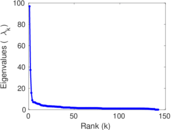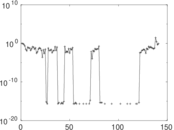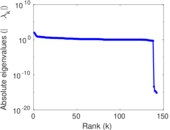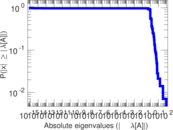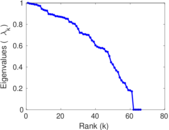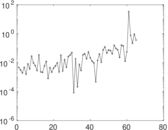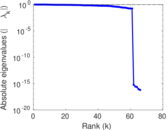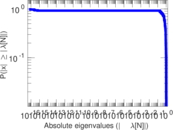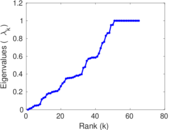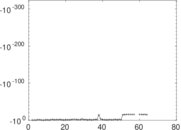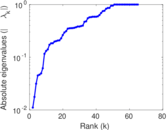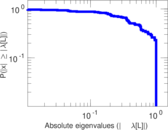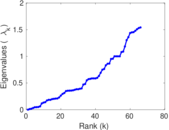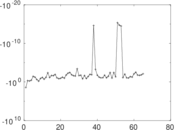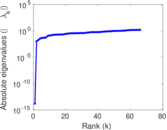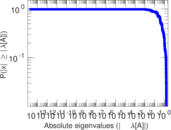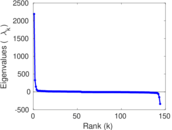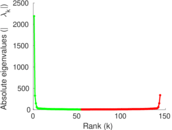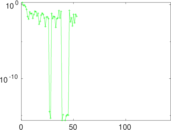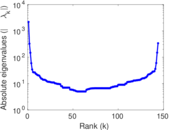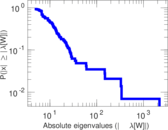# Wikiquote edits (su)

This is the bipartite edit network of the Sundanese Wikiquote. It contains users and pages from the Sundanese Wikiquote, connected by edit events. Each edge represents an edit. The dataset includes the timestamp of each edit.

 Code `qsu` Internal name `edit-suwikiquote` Name Wikiquote edits (su) Data source http://dumps.wikimedia.org/ AvailabilityDataset is available for download Consistency checkDataset passed all tests Category Authorship network Dataset timestamp 2017-10-20 Node meaning User, article Edge meaning Edit Network formatBipartite, undirected Edge typeUnweighted, multiple edges Temporal dataEdges are annotated with timestamps

## Statistics

 Size n = 2,184 Left size n1 = 144 Right size n2 = 2,040 Volume m = 3,029 Unique edge count m̿ = 2,153 Wedge count s = 1,255,311 Claw count z = 649,765,501 Cross count x = 254,820,916,742 Square count q = 353 4-Tour count T4 = 5,034,718 Maximum degree dmax = 2,287 Maximum left degree d1max = 2,287 Maximum right degree d2max = 48 Average degree d = 2.773 81 Average left degree d1 = 21.034 7 Average right degree d2 = 1.484 80 Fill p = 0.007 329 11 Average edge multiplicity m̃ = 1.406 87 Size of LCC N = 1,949 Diameter δ = 19 50-Percentile effective diameter δ0.5 = 1.651 83 90-Percentile effective diameter δ0.9 = 3.820 76 Median distance δM = 2 Mean distance δm = 2.656 66 Gini coefficient G = 0.638 908 Balanced inequality ratio P = 0.248 267 Left balanced inequality ratio P1 = 0.097 061 7 Right balanced inequality ratio P2 = 0.396 500 Relative edge distribution entropy Her = 0.684 947 Power law exponent γ = 13.301 6 Tail power law exponent γt = 3.731 00 Tail power law exponent with p γ3 = 3.731 00 p-value p = 0.000 00 Left tail power law exponent with p γ3,1 = 1.901 00 Left p-value p1 = 0.145 000 Right tail power law exponent with p γ3,2 = 4.561 00 Right p-value p2 = 0.439 000 Degree assortativity ρ = −0.393 363 Degree assortativity p-value pρ = 1.320 97 × 10−80 Spectral norm α = 96.862 6 Algebraic connectivity a = 0.011 078 8 Spectral separation |λ1[A] / λ2[A]| = 2.601 80 Controllability C = 1,887 Relative controllability Cr = 0.872 399

## Plots

### Fruchterman–Reingold graph drawing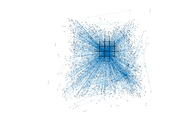### Degree distribution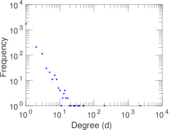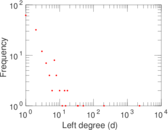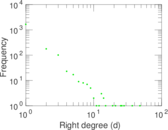### Cumulative degree distribution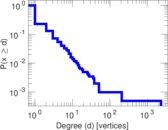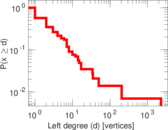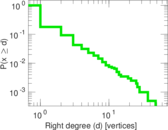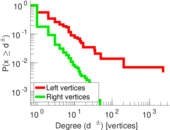### Lorenz curve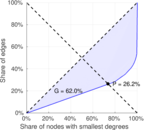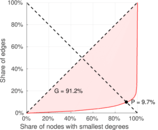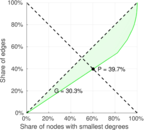### Spectral distribution of the adjacency matrix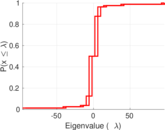### Spectral distribution of the normalized adjacency matrix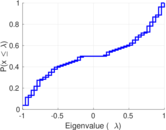### Spectral distribution of the Laplacian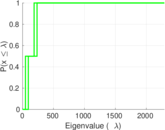### Spectral graph drawing based on the adjacency matrix### Spectral graph drawing based on the Laplacian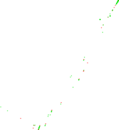### Spectral graph drawing based on the normalized adjacency matrix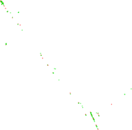### Degree assortativity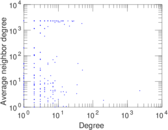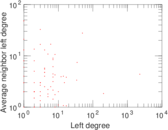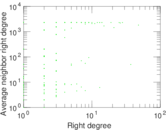### Zipf plot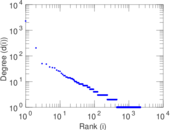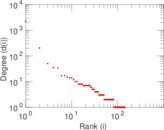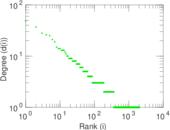### Hop distribution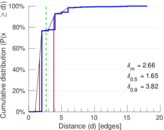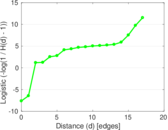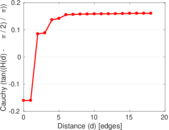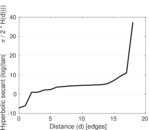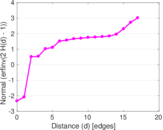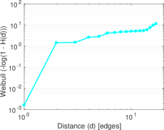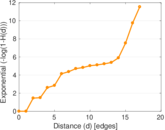### Double Laplacian graph drawing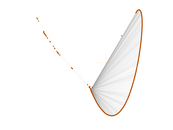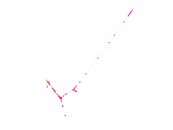### Delaunay graph drawing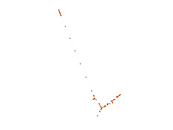### Edge weight/multiplicity distribution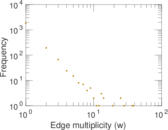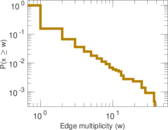### Temporal distribution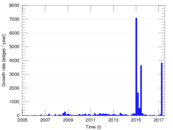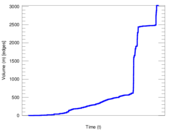### Temporal hop distribution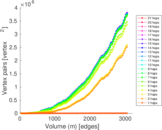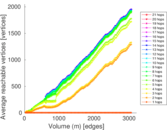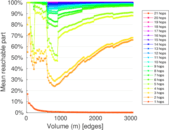### Diameter/density evolution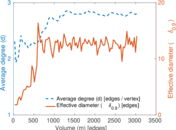### Matrix decompositions plots